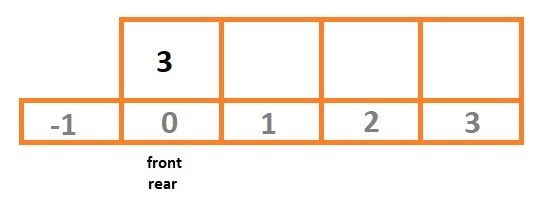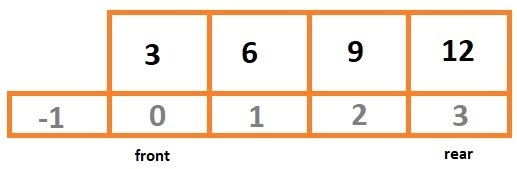# Implement dynamic Deque using templates class and a circular array

## Introduction

Deque or Double Ended Queue is a particular type of Queue where insertion and deletion are possible from two sides- front and rear. A deque is an ordered collection of items in which the capabilities of stacks and queues can be seen in a single data structure.

In this article, we will implement a dynamic deque using the templates class and a circular array.

## Problem Statement

Our task is to create a Dynamic Deque using the templates class and implement it using a circular array. The deque must have the following functions:

• dqCapacity(): returns the capacity of the deque. Here, capacity means the number of elements the deque can hold.
• dqRetSize(): return the number of elements in the deque.
• dqIsEmpty(): return whether our deque is empty or not.
• dqIsFull(): return whether our deque is full or not.
• DqPushFront(dQue): Pushes dQue at the front of the deque.
• dqPushRear(dQue): Pushes dQue at the end of the deque.
• dqPopFront(): Pops and return the rear element of the deque.
• dqPopRear(): Pops and returns the front element of the deque.
• dqFront(): Returns the front element of the deque.
• dqRear(): Returns the rear element of the deque.

## Example

1. Initially, our deque is empty and looks like this:

Capacity = 4

Size = 02. On inserting one element to the rear of our deque.

Capacity = 4

Size = 13. On inserting three more elements to the rear.
Capacity = 4
Size = 44. On inserting one element to the front of the deque.
Capacity = 8
Size = 5

5. On popping two elements from the rear.
Capacity = 8
Size = 3

6. On popping one element from the front.
Capacity = 8
Size = 2

## Approach and Explanation

• Our class DQ contains five private variables, which are:
• int frontIdx: stores the front index of the deque.
• int rearIdx: stores the rear index of the deque.
• DQ *dq: circular array to implement our dynamic deque.
• int dqSize: stores the size of our deque.
• int dqCap: stores the capacity of our deque, initialize to 4.

• Inside the constructor, we initialize the variables of our class as follows:
• We declare a new array of type DQ and size dqSize.
• We initialize the frontIdx and rearIdx to -1.
• We initialize the dqSize to 0.

• Now we create all the functions asked in the problem statement, one by one.

• int DeQue<DQ>:: dqCapacity(): returns the value of dqCap.

• int DeQue<DQ>:: dqRetSize(): return the value of dqSize.

• bool DeQue<DQ>:: dqIsEmpty(): if the front and rear index are -1 returns true, else false.

• bool DeQue<DQ>:: dqIsFull(): if the value of dqCap and dqSize is equal returns true, else false.

• DQ DeQue<DQ>:: dqFront(): if the deque is empty, the function displays “DeQue Underflow”. Else returns the element at dq[frontIdx].

• DQ DeQue<DQ>:: dqRear(): if the deque is empty, the function displays “DeQue Underflow”. Else returns the element at dq[rearIdx].

• void DeQue<DQ>:: dqPushFront(DQ dQue): It does the following three tasks:
• Checks if our deque dq is full or not. If the deque is full, we double its size by multiplying dqCap by two. Create a temporary array of type DQ and copy the elements of the old array into the new. Finally, deallocate the old array.
• If our deque DQ is empty, then set the front and rear index to 0, and push the element at dq[rearIdx].
• Else set the value of frontIdx to (frontIdx+1)%dqCap push the element at dq[rearIdx], increase the value of dqSize by one.

• void DeQue<DQ>:: dqPushRear(DQ dQue): It does the following three tasks:
• Checks if our deque dq is full or not. If the deque is full, we double its size by multiplying dqCap by two. Create a temporary array of type DQ and copy the elements of the old array into the new. Finally, deallocate the old array.
• If our deque DQ is empty, then set the front and rear index to 0, and push the element at dq[rearIdx].
• Else set the value of rearIdx to (rearIdx+1)%dqCap, push the element at dq[rearIdx], increase the value of dqSize by one.

• int DeQue<DQ>:: dqPopFront(): This function does the following three tasks:
• If our deque is empty, display “DeQue Underflow” and exit the function.
• If our deque has only one element, then store that element in a temporary variable, set the values of frontIdx and rearIdx to -1, decrease the value of dqSize by one, and return temp.
• Else store the element at dq[frontIdx], change the value of frontIdx to (frontIdx-1+dqCap)%dqCap, decrease the value of dqSize by one and return the stored element.

• int DeQue<DQ>:: dqPopRear(): This function does the following three tasks:
• If our deque is empty, display “DeQue Underflow” and exit the function.
• If our deque has only one element, then store that element in a temporary variable, set the values of frontIdx and rearIdx to -1, decrease the value of dqSize by one, and return temp.
• Else store the element at dq[rearIdx], change the value of rearIdx to (rearIdx-1+dqCap)%dqCap, decrease the value of dqSize by one and return the stored element.

## C++ implementation

``````#include<iostream>
#include<stdlib.h>
using namespace std;

template <class DQ>
class DeQue{

private:
int frontIdx;
int rearIdx;
DQ *dq;
int dqSize;
int dqCap = 4;

public:
DeQue(){
dq = new DQ[dqCap];
frontIdx = rearIdx = -1;
dqSize = 0;
}

int dqCapacity();
int dqRetSize();
bool dqIsEmpty();
bool dqIsFull();
void dqPushFront(DQ dQue);
void dqPushRear(DQ dQue);
int dqPopFront();
int dqPopRear();
DQ dqFront();
DQ dqRear();
};

template <class DQ>
int DeQue<DQ>::dqCapacity(){
return dqCap;
}

template <class DQ>
int DeQue<DQ>::dqRetSize(){
return dqSize;
}

template <class DQ>
bool DeQue<DQ>::dqIsEmpty(){
if(frontIdx == -1 && rearIdx == -1){
return true;
}else{
return false;
}
}

template <class DQ>
bool DeQue<DQ>::dqIsFull(){
if(dqCap == dqSize){
return true;
}else{
return false;
}
}

template <class DQ>
DQ DeQue<DQ>::dqFront(){
if(dqIsEmpty()){
cout << "DeQue Underflow" << endl;
abort();
}

return dq[frontIdx];
}

template <class DQ>
DQ DeQue<DQ>::dqRear(){
if(dqIsEmpty()){
cout << "DeQue Underflow" << endl;
abort();
}

return dq[rearIdx];
}

template<class DQ>
void DeQue<DQ>::dqPushFront(DQ dQue){

if(dqIsFull()){
dqCap = dqCap*2;

DQ *temp = new DQ[dqCap];

int i = frontIdx, j=0;

while(i != rearIdx){
temp[j++] = dq[i];
i = (i+1) % dqSize;
}

temp[j] = dq[i];

frontIdx = 0;
rearIdx = dqSize - 1;

delete[] dq;
dq = temp;
}

if(dqIsEmpty()){
frontIdx = rearIdx = 0;
dq[rearIdx] = dQue;
dqSize++;
return;
}

frontIdx = (frontIdx - 1 + dqCap) % dqCap;
dq[frontIdx] = dQue;
dqSize++;
return;
}

template <class DQ>
void DeQue<DQ>::dqPushRear(DQ dQue){

if(dqIsFull()){
dqCap = dqCap * 2;

DQ *temp = new DQ[dqCap];

int i = frontIdx, j=0;

while(i != rearIdx){
temp[j++] = dq[i];
i = (i + 1) % dqSize;
}

temp[j] = dq[i];

frontIdx = 0;
rearIdx = dqSize-1;

delete[] dq;
dq = temp;
}

if(dqIsEmpty()){
frontIdx = rearIdx = 0;
dq[rearIdx] = dQue;
dqSize++;
return;
}

rearIdx = (rearIdx + 1) % dqCap;
dq[rearIdx] = dQue;
dqSize++;
return;
}

template <class DQ>
int DeQue<DQ>::dqPopFront(){

int temp;

if(dqIsEmpty()){
cout << "DeQue Underflow" << endl;
abort();
}

if(frontIdx == rearIdx){
temp = dq[frontIdx];
frontIdx = rearIdx = -1;
dqSize--;
return temp;
}

temp = dq[frontIdx];
frontIdx = (frontIdx + 1) % dqCap;
dqSize--;
return temp;
}

template <class DQ>
int DeQue<DQ>::dqPopRear(){

int temp;

if(dqIsEmpty()){
cout << "DeQue Underflow" << endl;
abort();
}

if(frontIdx == rearIdx){
temp = dq[rearIdx];
frontIdx = rearIdx = -1;
dqSize--;
return temp;
}

temp = dq[rearIdx];
rearIdx = (rearIdx - 1 + dqCap) % dqCap;
dqSize--;
return temp;
}

int main(){
DeQue<int> circ_q;

cout << endl << "*****AT EMPTY DEQUE STATE*****" << endl;
cout << "Capacity of the Circular Queue:" << circ_q.dqCapacity() << endl;
cout << "Size of the Circular Queue: " << circ_q.dqRetSize() << endl;

circ_q.dqPushRear(3);
circ_q.dqPushRear(9);
circ_q.dqPushRear(12);
circ_q.dqPushRear(15);
circ_q.dqPushRear(18);

circ_q.dqPushFront(2);
circ_q.dqPushFront(4);
circ_q.dqPushFront(6);
circ_q.dqPushFront(8);
circ_q.dqPushFront(10);

cout << endl <<"*****AFTER INSERTION INTO DEQUE*****" << endl;
cout << "Capacity of the Circular Queue:" << circ_q.dqCapacity() << endl;
cout << "Size of the Circular Queue: " << circ_q.dqRetSize() << endl;
cout << "Front element is: " << circ_q.dqFront() << endl;
cout << "Rear element is: " << circ_q.dqRear() << endl;

cout << endl << "POPPING FRONT ELEMENT" << endl ;
cout << circ_q.dqPopFront() << " SUCCESSFULLY POPPED FROM FRONT"<< endl;
cout << circ_q.dqPopFront() << " SUCCESSFULLY POPPED FROM FRONT"<< endl;

cout << "POPPING REAR ELEMENT" << endl;
cout << circ_q.dqPopRear() << " SUCCESSFULLY POPPED FROM REAR"<< endl;

cout << endl;

cout << "*****AFTER CHANGES*****" << endl;
cout << "Capacity of the Circular Queue:" << circ_q.dqCapacity() << endl;
cout << "Size of the Circular Queue: " << circ_q.dqRetSize() << endl;
cout << "Front element is: " << circ_q.dqFront() << endl;
cout << "Rear element is: " << circ_q.dqRear() << endl;

return 0;
}``````

OUTPUT:

``````*****AT EMPTY DEQUE STATE*****
Capacity of the Circular Queue:4
Size of the Circular Queue: 0

*****AFTER INSERTION INTO DEQUE*****
Capacity of the Circular Queue:16
Size of the Circular Queue: 10
Front element is: 10
Rear element is: 18

POPPING FRONT ELEMENT
10 SUCCESSFULLY POPPED FROM FRONT
8 SUCCESSFULLY POPPED FROM FRONT
POPPING REAR ELEMENT
18 SUCCESSFULLY POPPED FROM REAR

*****AFTER CHANGES*****
Capacity of the Circular Queue:16
Size of the Circular Queue: 7
Front element is: 6
Rear element is: 15
``````

## Complexities

### Time Complexity

The time complexity of the given implementation is O(N) since every time we double the deque, we copy all of its elements into the new deque. Thus,

T(n) = O(N)

### Space Complexity

The space complexity of the given implementation is O(N). This is the extra space used by the program while copying the deque elements. Thus,

Space Complexity = O(N)

1. What are the other ways of implementing Deque?
Ans. We can use a doubly-linked list to implement Deque. In that case, we will have to maintain the front and rear pointers.

2. What is the application of a Deque?
Ans. A deque can be used in the work-stealing algorithm. This algorithm is used for task scheduling for several processors.

## Key Takeaways

To summarize the article, we discussed implementing dynamic deque using the templates class and a circular array. We saw the problem statement, an example, the approach of the problem, along with its explanation. We saw the solution code and output along with the time and space complexities.

Improve your coding skills by practicing various problems of various difficulty levels on our CodeStudio.

Learn about various topics like Web Technologies, Programing Fundamentals, Aptitude, DSA, and much more from our CN Library | Free Online Coding Resources.

Happy Coding!